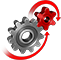# WolframSystemModeler

## Balancing Ball

This model studies a ball rolling on top of a beam. The ball translational acceleration will be dependent on how the beam is angled. In this example, two different control schemes are studied, the PID regulator and the LQ regulator, which can be used to control the position of the ball along the beam, using the beam angle as input.

To run this example, you'll need

### The Model

Gravity acts on the ball, providing a downward force that is proportional to the mass of the object. When the beam is perpendicular to the gravitational force, parallel to the ground, the normal force exactly cancels out the gravitational force and no acceleration is applied to the ball. By carefully setting the angle of the beam, the position of the ball along the beam can be controlled. The model can be used to try out different methods for controlling the position of the ball, both in simulation and with real-time connection to hardware.The angle of the beam is controlled precisely by a servo. On either side of the beam is a bumper that will stop the ball from rolling off of the beam. The model contains multibody objects onto which custom CAD models have been loaded to visualize the system.

### Visualization

Since the model is built in SystemModeler using the MultiBody library, an animation of the model is generated automatically. Custom CAD models have been loaded onto the objects to give an accurate representation of the real-world system.
Output from the model is used to control the real-world system, and input from the real-world system is used to animate the model.

### Controller Design

Use the Wolfram Language together with SystemModeler to design a controller for the hardware, based on the model.The Wolfram Language can automatically linearize system models, which can in turn be used for pole placement for PID controllers and calculate gains for LQ regulators.With the controllers constructed, you can easily connect the controller to either the software model or custom hardware, using the ModelPlug library, and compare how the controllers perform.

Design & Compare

Use the Wolfram Language to design controllers and compare their output.